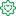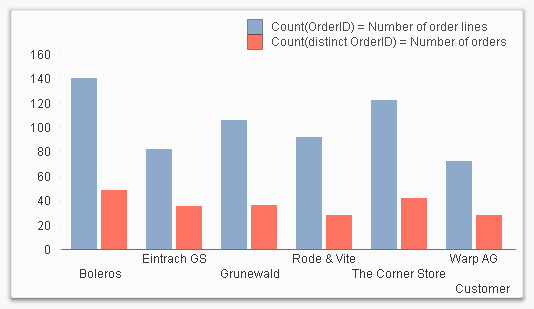Announcements
The way to achieve your own success is the willingness to help somebody else. Go for it!Former Employee

## Count or Count distinct?

When counting something in QlikView, you should use the Count() aggregation function. But – should you use Count or Count distinct?

Although very similar, the two constructions return completely different results. And often the wrong one is used…

So, let me start by clarifying what the two constructions really count:

• Count( <expression> ) counts the number of records in the data.
• Count( distinct <expression> ) counts the number of distinct values of the expression.

Only instances where the expression isn't NULL are included. Further, if the expression contains references to more than one field, an n-tuple (a temporary table) is created from the cross product of the constituent fields, and the count is performed in the n-tuple instead of in the data table.

This means that in the common case – when the <expression> is a simple field reference – the Count(…) is equivalent to the number of rows in the data table, whereas the Count(distinct …) is equivalent to the number of rows in the symbol table. Read more about data tables and symbol tables here.

An example: You have a fact table and you want to count the number of orders. Then Count(OrderID) will return the number of records in the fact table, which often is a number larger than the number of orders, since one order usually can have several order lines. In this case you probably want to count the number of unique Order IDs, and should most likely choose Count(distinct OrderID) instead.

So, Count(OrderID) does not count the orders!Another trap I have seen people fall into, is when a key field is used as parameter in the Count() function. If the distinct clause is used, there is no problem: The number of distinct values is well defined also for key fields. But if you omit the distinct clause, the number is not well defined: In which data table should QlikView count the number of records? There are several possible source tables, since the field is a key.

The Count(Key) will however return a number, but this number is not always the number you want. Sometimes it will be the count from the "wrong" table. So I would recommend that you never use a Count() function on a key field, unless you use the distinct clause.

Otherwise, the work-around for using the Count() function (without the distinct clause) on a key field, is the create a copy of this field in the table where you want to count the records, and use this field inside the Count() instead of the key field.

Bottom line: For key fields, either use the distinct clause, or the above work-around.

HIC